.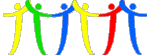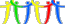HOT ISSUES

ECON DEVELOPMT CORP

WATER  QUALITY

SOLICITORS AT DOORS

MARCELLUS SHALE/Fracking

PESTICIDE SPRAYING

WAWA NEAR BAEDER

RED LIGHT CAMERAS

VOTING- ELECTIONS

ST.MICHAEL'S

POLICE MATTTERS
MANOR WOODS
GALMAN Near LENOX RD
FLOODING
BILLBOARDS
COMMERCIALS ON TV
NOBLE TRAIN AREA
BAEDERWOOD SHOP CNTR
FAIRWAY TRANSIT DISTRICT
ZONING REWRITES
ZONING VARIANCES
SCHOOL SUPERINTENDENT
WHERE TO FIND LAWS AND REGS
ANTI-DISCRIMINATION ORDINANCE
MCDONALDS- DUNKIN DONUTS
A PLACE TO MEET
TAX COLLECTION COSTS
YORK RD CORRIDOR PROJEct
ILLEGAL ALIENS
E-VERIFY PROGRAMand more...
LOST AND FOUND
THE BUDGET PROCESS
THE TAX PROCESS
TAX ABATEMENTS
ZONING &
DEVELOPMENT
HUNTER SOCCER CLUBHOUSE
LORIMOR PONDS ESTATES
RYDAL WATERS
EMINENT DOMAIN IN ROSLYN
TV ACCESS CHANNELS
CORRIDOR REVITALIZATION
WILLIARD COMPLEX IN GLENSIDE
OPEN SPACE
ARDSLEY WILDLIFE SANCTUARY
ENVIRONMENTAL ISSUES
DEER HUNTS IN TOWNSHIP
CELL PHONE TOWERS
CONTRACTORS
TRASH - PAY TO THROW
LITTER
SCHOOL ISSUES
JOBS FOR OUR KIDS
ANONYMOUS REPORTING
TOOLS FOR RESIDENTS LIKE
WHERE TOFIND LAWS AND REGSand more...

COMMUNITY GROUPS
ACTIVITIES -EVENTS
TOWNSHIP GOVERNMENTand more...

SITE MAP
POLICIES
PRIVACY
DISCLAIMER
REPORT ABUSES
CORRECT AN ERRORRegarding Postings:
All views
Pro and Con
including
multiple views
on either side
will be given
equal access
on this site.The Abington Citizens Network where Abington, PA residents can share ideas and join forces to build a better community 1209 Rydal RdTHE NUTSHELL   : This is about a proposal to take a 7.79 + acre lot - at least partially on a slope, that could at most support 7 single family homes under the current R 1 zoning, and to ask the Commissioners  to violate the rights and wishes of the residents and allow this developer to change the zoning in order to build  either 18 Townhouse units and 16 Duplex units (34 units total)  where only 7 should currently be  .... or maybe a mix of Townhouse condo's, Duplex condos and single family homes  for even  more than 34 units ( again, where only 6 more single family homes should be, if current laws codes were upheld. )   This will increase the bottom line of the  developer and undoubtedly detract from the value of the beautiful homes that are currently there.  The developer's wealth would come at the expense of the citizens' rights to have their zoning protected.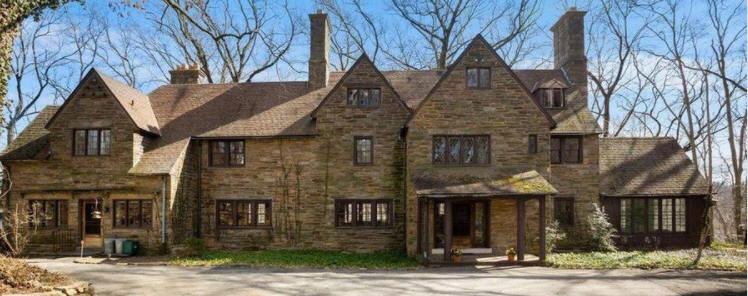))))))))))))))))))))))))))))))))))))))))))))))))))))))))))))))))))))))))))))))))))))ERRORS? Please check all details  for accuracy . If there are discrepancies please let us know so we can correct anything that needs correcting.    ))))))))))))))))))))))))))))))))))))))))))))))))))))))))))))))))))))))))))))))))))))ACTION NEEDED:  After reading this - please connect in with the neighbors who are  keeping all informed of meetings,   holding discussions, lending their voices to the legislators and taking action.Sign the petition   or  Email lel@abingtoncitizens.com to be connected in ))))))))))))))))))))))))))))))))))))))))))))))))))))))))))))))))))))))))))))))))))))1209 RYDAL ROAD    View the Property here   https://www.redfin.com/PA/Rydal/1209-Rydal-Rd-19046/home/38247017   ))))))))))))))))))))))))))))))))))))))))))))))))))))))))))))))))))))))))))))))))))))VALUE:  It is  off  the market now,  but before Chaverim bought it, it was listed  at well over \$1M at one point  From Zillow  10-21:  Zillow estimates this 5 bd 6.5 bth  4947 sf  home at   \$1,403,600   https://www.zillow.com/homedetails/1209-Rydal-Rd-Rydal-PA-19046/9905064_zpid/ So why did it sell at \$600K? Yes, it needs work - but THAT much? Almost a million? ))))))))))))))))))))))))))))))))))))))))))))))))))))))))))))))))))))))))))))))))))))VALUE:  The assesssed value is \$680,050  and the Common Level Multiplier is 2.24  indicating  a potential  calculation of market value as \$1.52 M  So why did it sell at \$600K? ))))))))))))))))))))))))))))))))))))))))))))))))))))))))))))))))))))))))))))))))))))6-5-21 OWNER  CHAVERIM  CORPORATE PRESENCE IS BORN June 5 , 2019 Chaverim Realty Partners LLC  4122  Haverford Ave  Suite 11  Phila Pa 19104 The Registered Agent was Active Realty 1751 Easton Road Willow Grove PA 19090 Montgomery   David Mermelstein Pres,  Solomon Mermelstein  Sec & Treas ))))))))))))))))))))))))))))))))))))))))))))))))))))))))))))))))))))))))))))))))))))6-27-19  CHAVERIM PURCHASES THE PLOT  June  27, 2019  for   \$600,000 per Montco Property Records Sale Date        27-JUN-19      Sale Price        \$600,000     Tax Stamps     6000    Deed Book and Page  6143-02991 Grantor            STEINBERG MARY ELLEN        Grantee           CHAVERIM REALTY PARTNERS LLCDate Recorded 10-JUL-19     Sale Date        27-JUN-19 )))))))))))))))))))))))))))))))))))))))))))))))))))))))))))))))))))))))))))))))))))) he buidling contractor  MB Crafters   Moshe Barazani  The business is registered on Ambler Rd in Abington on at least one report - but the principal Moshe Barazani, coincidentally ives just a few blocks away from Commissioner Hecker. They are listed with 2 employees and annual revenue just over \$300K . There is not date on that report - but it came up on D&B  in mid October 2021 https://www.dnb.com/business-directory/company-profiles.m_b_crafters_of_pa_inc.fce627345a13b542bfa2724667ce85ff.html ))))))))))))))))))))))))))))))))))))))))))))))))))))))))))))))))))))))))))))))))))))))8-9-21  CHAVERIM'S BUILDING CONTRACTOR MB CONTRACTORS  MEETS WITH RESIDENTS Aug 9, 2021  Craig Lerch ( realtor involved in the sale) introduces MB Crafters , the building contractor .  They offer their proposal  for feedback. There is some confusion over the exact realtionships - for instance , whether  MB Crafters is just the Builder/Contractor -or partner .  but we are trying to clear that up.  Chaverim Partners is the only entity  listed in the Montgomery County property records as the owner. ))))))))))))))))))))))))))))))))))))))))))))))))))))))))))))))))))))))))))))))))TOWNSHIP ENCOURAGEMENT TO VIOLATE ZONING ?  We are also seeking to clear up who it was in the Township that encouraged denser development  be offered .  Some stories have the Commissioner saying that is not true - others have him syaing that he will find out who it was .  SThere may have been a  zoom call with the Township where the deeper density was encouraged.  All that is yet to be sorted out.  Please let me know if you have learned more about this, so we can keep this page as accurate as possible.  ))))))))))))))))))))))))))))))))))))))))))))))))))))))))))))))))))))))))))))))))))))A SECTION FROM THE PLANS as presented  8-9-21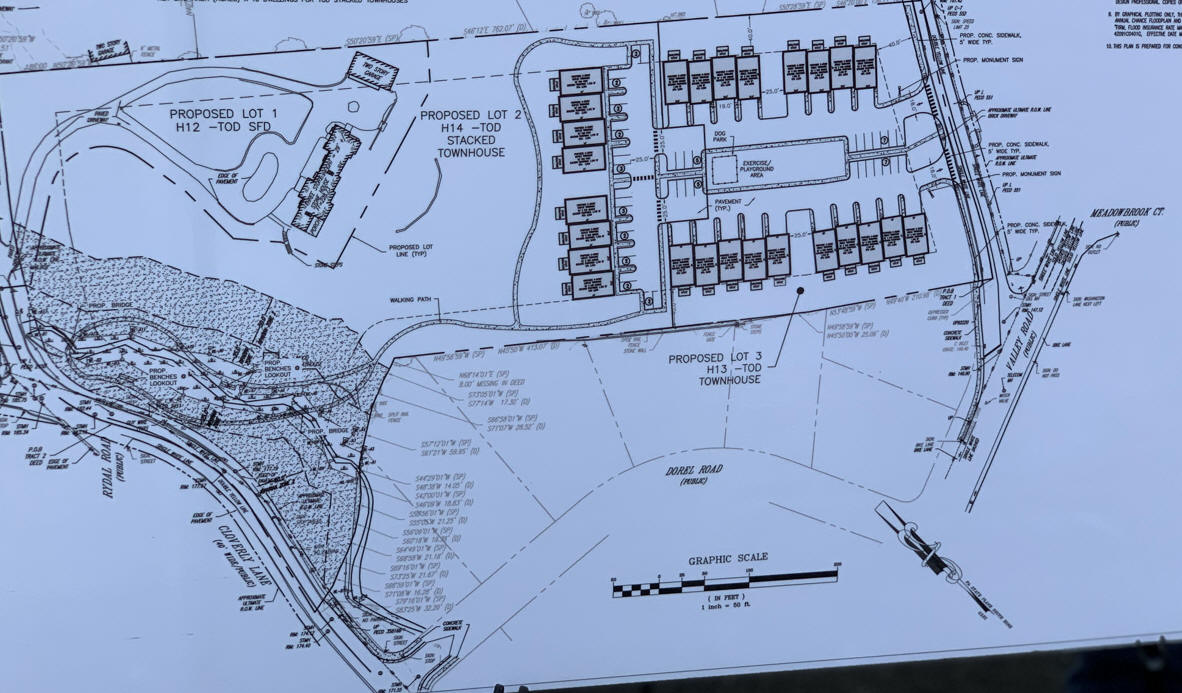))))))))))))))))))))))))))))))))))))))))))))))))))))))))))))))))))))))))))))))))))))PART OF THE "TOD CHART" AS PRESENTED  8-9-21 TOD stands for Transit Oriented Development - and generally there are monies available to builders to build densely around Transit hubs - like the Rydal Train station. From our pockets to theirs - to do things we don't want done.Yes - the TOD funds come from taxes you pay.  Or, as they would have you believe, from the tooth fairy.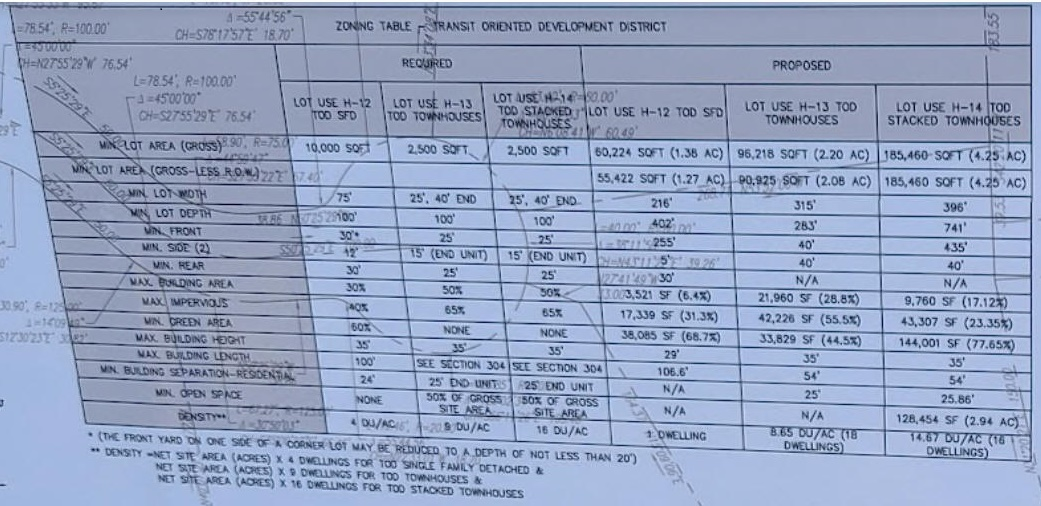))))))))))))))))))))))))))))))))))))))))))))))))))))))))))))))))))))))))))))))))))))THE PROPOSED HOUSING INFO below is from the bottom of the chart shown above - It shows 4 single family homes, 9 townhouses and 16 stacked townhouse duplexes... but  SOME residents came away with the sense that 18 Townhouses and 16 Duplexes were what was in the plan. Others thought thtere was a mix like this.  Some understood all to be condos. So as of this 10-21 writing, confusion reigns.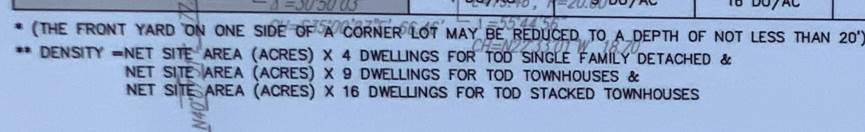))))))))))))))))))))))))))))))))))))))))))))))))))))))))))))))))))))))))))))))))))))The Plot in relation to its neighbors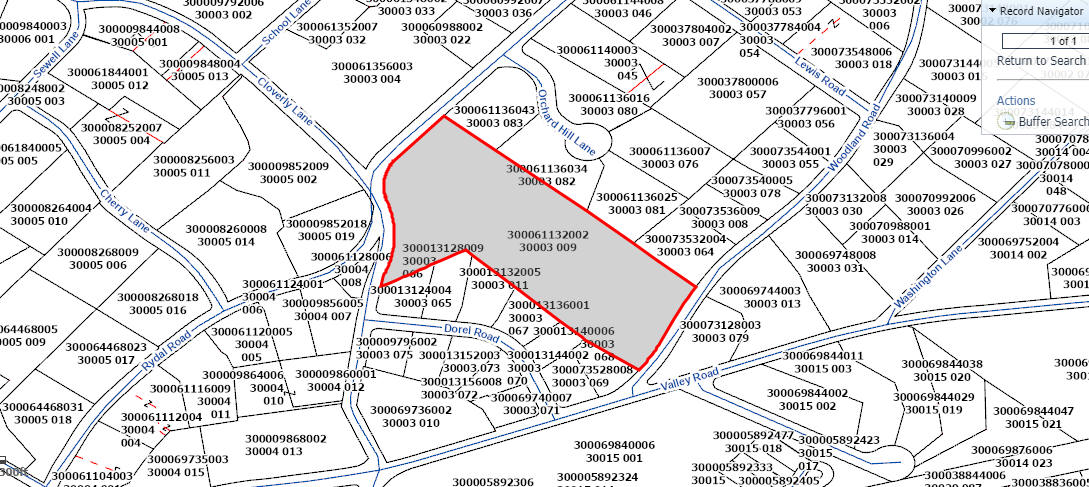))))))))))))))))))))))))))))))))))))))))))))))))))))))))))))))))))))))))))))))))))))The plot in relation to the train  station - Where is the walkable area? Who will pay for the sidewalks ? Who will maintain them ? The intersection at Valley and Susquehann is crazy busy at rush hour . Is it smart to drive pedestrian traffic there . It is NOT for the benefit of the  current residents who voted these legislators in to oversee their interests. Any benefit will go to the developer and to new tenants or owners of these residences .... at the expense of the others already there.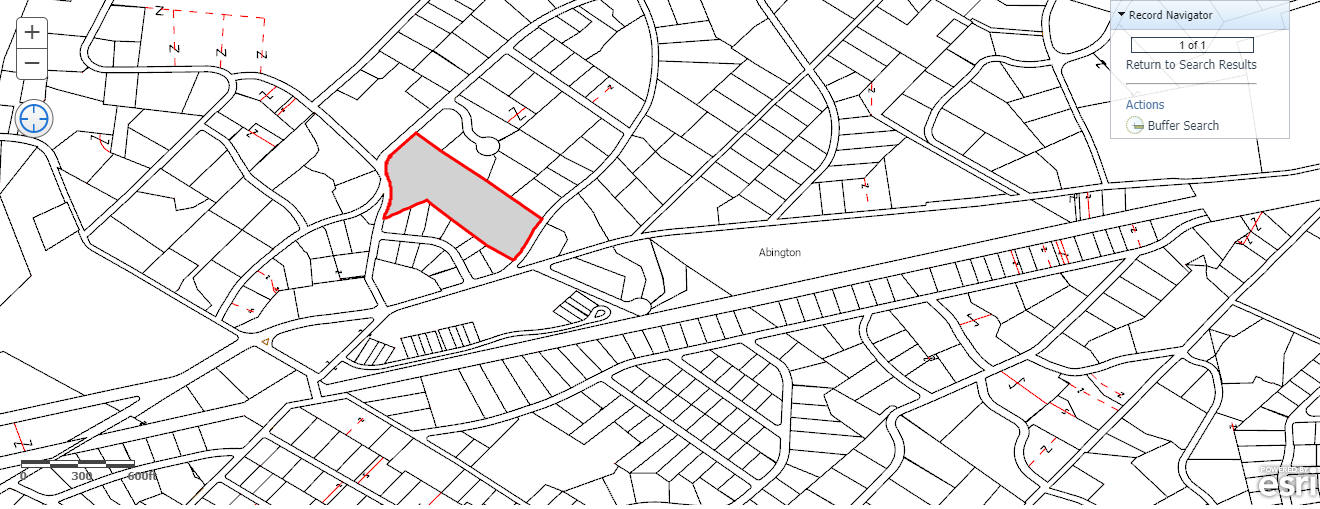))))))))))))))))))))))))))))))))))))))))))))))))))))))))))))))))))))))))))))))))))))UNDERSTANDING SPOT ZONING  and your disappearing zoning rights. You cannot simply request to have your own property improved for your benefit alone by changing the zoning for yourself, and not for others that have the same kind of properties. So when developers add things into our Zoning Code to benefit themselves, often other properties get rights that the residents near the other properties never even learn about and never have a say in - even though the law intends that they should. Our Township famously does not reveal these things or explain them to the residents impacted. ### When the York Rd Wawa  at the top of the Fairway got their massive benefits ( the equivalent of 23 variances or waivers), other properties were affected and would now ALSO be allowed to have a gas station and 24 hour  operation.  Contiguous neighbors to these other properties, however,  were not alerted.  ### When the former YMCA property got their new rights as a gigantic gift from the Commissioners, significant changes to other properties occurred. The impact of that was never explored. ### Penn State just held many meetings about changes they soon hope to have, without notifying the neighbors near other properties that may get the same uses by right.  Manor College will also change and those neighbors were never given any proper explanation. The same is likely to happen here. Spot Zoning,  on it's face, is improper and illegal - but the laws and codes are being twisted or ignored in unethical ways  that leave residents helpless unless they want to dip into their own pockets and try to sue either a wealthy developer or a Township that has immunity. Recent case law twisted the intents even further by suggesting if the Commissioners WANT that kind of zoning - if it is in theri "vision" then it is not spot zoning.  )))))))))))))))))))))))))))))))))))))))))))))))))))))))))))))))))))))))))))))))))))) Now - as of 10-14-21 we have another threat to our zoning .  The Township revealed at the Committee of the Whole on 10-14-21 that they are planning to unveil an even further erosion of your zoning rights. They want to give themselves the right to "amend " the zoning ordinance virtually at will.  That means you would no longer have any strong zoning protections for your property at all.  They tried to sneak this into a paragraph some years ago that would have affected  most of the York Rd corridor .  The simple phrase was discovered and there was an outcry to remove it .  Now they put the assault on your zoning rights into an agenda item that was billed as Public Comment changes  --- and it would affect the entire Township.  More to come on this matter.       Whether our laws and codes work properly or don't is in the hands of your legislators (even though they falsely may tell you it is not ) .  Do not allow them to continue passing legislation that removes your rights  and puts everything in their hands. Hold them accountable by learning  what they are doing - but protecting your public comment rights so you can inform others - and by learning how your system of elections works, so you can find new candidates to replace those that are "hard of hearing".  ))))))))))))))))))))))))))))))))))))))))))))))))))))))))))))))))))))))))))))))))))))  UNDERSTANDING MAP AND TEXT AMENDMENTS  Zoning Hearing Boards hearings are quasi-judicial & have very specific processes and laws that must be followed. They have very specific notification rules.   Text and map amendments, by contrast, are the Wild West of  zoning changes  and have almost zero rules ... no notifications required until the hearing - (you may  have missed the earlier 11 meetings... they don't care ) , no required process of review and nothing to inhibit 8 of our 15 Commissioners from saying AYE even when the entire matter isn't even remotely in your interest.  This should be a concern to all..... 8 individuals are not had to "entice" into an action that benefits a developer. This project, itself, has even started out with  Township actions favoring the developer rather than the residents served.   ))))))))))))))))))))))))))))))))))))))))))))))))))))))))))))))))))))))))))))))))))))This will likely be a text and map amendment proposal   ----  not a Zoning Hearing Board  matter - which hears far less dramatic changes to zoning than this. The Zoning Hearing Board  handles variances and waivers, etc.  perhaps to extend further out the side..... or build a deck - or increase the property footprint.  And variances need a hardship. There would be no reasonable hardship here for this kind of monumental change. Unless greed is now a hardship.   I remain willing  to admit if I've made a bad call here, but I am pretty darn sure the only way to accomplish this is with a map amendment, or a text and map amendment.....meaning that they intend to change the zoning map  ( see the paragraph below this ) to a new zoning designation that allows all that they want --- or if not just a map amendment ,  they  will add  new text into the current or new zoning designation to allow what they want . Such a  change  would impact all other R1 properties with similar conditions and could be a disaster . The extent of the changes is never explained. The Commissioners don't require it , however unethical that may be.   They just tried to pull a "fast one" like this over at  Penn State which would have changed the R1 zoning in multiple places, if not Township-wide.    ))))))))))))))))))))))))))))))))))))))))))))))))))))))))))))))))))))))))))))))))))))HERE IS YOUR ZONING CODE -  near the very end are the uses that are already allowed in the R1 districtand the zoning maps      Remember : whatever they put in it will apply to all other properties that meet the same specs Township-wide. )))))))))))))))))))))))))))))))))))))))))))))))))))))))))))))))))))))))))))))))))))) On 10-19-21 there was a Comprehensive Plan meeting  with a focus this session on Future Land Use .  The Land use map tell the whole story. The blue dotted lines show the  1/2 mi  TOD's ( Transit Oriented Districts)  - ( see the 1/2 mile around the Rydal Station)  where dense development generally is  encouraged . The TOD around  Rydal Station includes 1209 Rydal Rd --- apparently they didn't want to wait for public input and the hearing and the plan to be officially passed.  The dense development already IS  "encouraged" without the input of the public. But the gray circles are  1/2 mi "Mixed Residential Overlay" areas.  I would interpret Mixed Use residential to be exactly what was proposed here --- but I am unsure how they are differentiaing their TOD overlay from the  "Mixed Use Residential Overlay". Does the TOD allow mixed use including commercial as TODs often do ?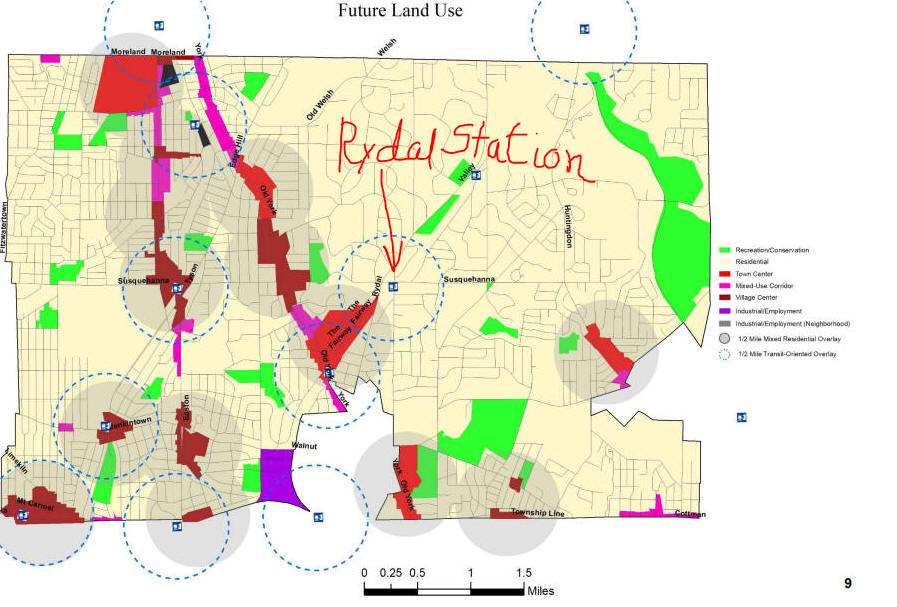)))))))))))))))))))))))))))))))))))))))))))))))))))))))))))))))))))))))))))))))))))IS THIS IN THE INTEREST OF THE HEALTH, SAFETY OR WELFARE OF THE RESIDENTS THESE COMMISSIONERS ARE SERVING ? No. Really, it is not.  Despite that it is the Commissioners'  duty to protect our welfare, this is contrary to that.  Multiple projects are, at this very moment,  being pushed through with no proper financial analysis  nor any analysis whatsoever of any kind that shows a  benefit to the current residents of the Township or shows the full extent of the negative impacts. Some of these impacts may even be very costly, may  devalue our homes, crowd our schools, raise our taxes,  keep us sitting in  time-wasting, pollution-creating  traffic and may require expansion of major facilities. Not to mention  changing the very character of our beautiful residential neighborhoods, or showing any recognition that  a great majority of residents again and again have vocalized that they do not want to be "urbanized".  And you should be sure to factor in  that your Commissioner is not the only one who votes on this issue .  You can find all the Commissioners here . Please let them hear from you. And please send their responses here so that others can know what they are saying about all of this. ))))))))))))))))))))))))))))))))))))))))))))))))))))))))))))))))))))))))))))))))))))Please join those holding discussions, lending their voices and taking action. Email lel@abingtoncitizens.com to be connected in . )))))))))))))))))))))))))))))))))))))))))))))))))))))))))))))))))))))))))))))))))))) __________We welcome your comments  to share either anonymously or with your name attached with your  fellow Abington residents.   Send  any updated information, comments or questions  to:THE NEWSLOOP   Sign up  here to receive our periodic Newsloop updates on issues that matter to us all       Knowledge is power. Stay informed to help shape your community & make a difference.   Abington Township revamped their entire website at the end of 2015. All links directed to the old site were broken. We will be reinstating links as we find them If the data is still available. Please let us know if you find a broken link. Send us the URL of the link and the Name of the page it is on, and if we can reinstate it, we will . DISCLAIMER: The information on this page or in this site may have unintentional inaccuracies, and also has opinions. It should not be relied upon as fact until investigated personally by the reader.  Please read our full  Disclaimer and read our Policies page before using this site.  All who find inaccuracies are asked to please contact us so we may correct them.h